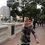# Algebra Set

Hi! This week's set consists in three problems in algebra that seem nice. I'm using the word "seem" because I've been working on them and haven't been able to solve them. Anyway, enjoy the problems, and, if you find them nice and/or fun, please reshare the note, so more people will be able to see the problems and try to tackle these hard problems. Just as a reference, problem 1 was taken from the Colombian book "Un recorrido por el álgebra" and problems 2 and 3 were taken from the first Costa Rican IMO TST 2014.

1. Find all functions $f:\mathbb{Z}^+\rightarrow\mathbb{R}$ such that $f(n+m)+f(n-m)=f(3n)$ for every $n,m\in{\mathbb{Z}^+}, n>m$.

2. Find all real solutions $(x_1,...,x_n)$ of the equation $(1-x_1)^2+(x_1-x_2)^2+...+(x_{n-1}-x_n)^2+x_n^2=\dfrac{1}{n+1}$

3. Let $x, y$ be real numbers such that $y>0$ and $7x^2+3xy+3y^2=1$. Show that $\dfrac{x^2+y^2}{y}\ge{\dfrac{1}{2}}$Note by José Marín Guzmán
6 years, 1 month ago

This discussion board is a place to discuss our Daily Challenges and the math and science related to those challenges. Explanations are more than just a solution — they should explain the steps and thinking strategies that you used to obtain the solution. Comments should further the discussion of math and science.

When posting on Brilliant:

• Use the emojis to react to an explanation, whether you're congratulating a job well done , or just really confused .
• Ask specific questions about the challenge or the steps in somebody's explanation. Well-posed questions can add a lot to the discussion, but posting "I don't understand!" doesn't help anyone.
• Try to contribute something new to the discussion, whether it is an extension, generalization or other idea related to the challenge.

MarkdownAppears as
*italics* or _italics_ italics
**bold** or __bold__ bold
- bulleted- list
• bulleted
• list
1. numbered2. list
1. numbered
2. list
Note: you must add a full line of space before and after lists for them to show up correctly
paragraph 1paragraph 2

paragraph 1

paragraph 2

[example link](https://brilliant.org)example link
> This is a quote
This is a quote
    # I indented these lines
# 4 spaces, and now they show
# up as a code block.

print "hello world"
# I indented these lines
# 4 spaces, and now they show
# up as a code block.

print "hello world"
MathAppears as
Remember to wrap math in $$ ... $$ or $ ... $ to ensure proper formatting.
2 \times 3 $2 \times 3$
2^{34} $2^{34}$
a_{i-1} $a_{i-1}$
\frac{2}{3} $\frac{2}{3}$
\sqrt{2} $\sqrt{2}$
\sum_{i=1}^3 $\sum_{i=1}^3$
\sin \theta $\sin \theta$
\boxed{123} $\boxed{123}$

Sort by:

For the second one:

Using the Cauchy-Schwarz Inequality we can write, $\displaystyle \begin{array}{c}\\ \bigg( (1-x_1)^2 + (x_1 - x_2 )^2 +\dotsb + (x_{n-1} - x_n)^2 + x_n^2 \bigg) \bigg( 1^2 + 1^2 + \dotsb + 1^2 + 1^2 \bigg) \geq & \\ \bigg( (1-x_1) + (x_1 - x_2 ) +\dotsb + (x_{n-1} - x_n)+ x_n \bigg)^2 & \\ \end{array}$

$\displaystyle \Rightarrow \Big( (1-x_1)^2 + (x_1 - x_2 )^2 +\dotsb + (x_{n-1} - x_n)^2 + x_n^2 \Big) (n+1) \geq ( 1)^2$ $\displaystyle \Rightarrow (1-x_1)^2 + (x_1 - x_2 )^2 +\dotsb + (x_{n-1} - x_n)^2 + x_n^2 \geq \frac{1}{n+1}$

But it is given that the equality condition holds true. Therefore, from the condition of equality in Cauchy-Schwarz, we obtain,
$\displaystyle \frac{1-x_1}{1} = \frac{x_1 - x_2}{1} = \dots = \frac{x_{n-1} - x_n}{1} = \frac{x_n}{1}$ $\displaystyle \Rightarrow x_{n-1} = 2x_n \text{ , } x_{n-2} = 3x_n ,\dotsc, \text{ } x_2 = (n-1)x_n \text{ and } x_1 = nx_n$.
Also, $\displaystyle 1 = x_1 + x_n \Rightarrow (n+1)x_n = 1 \Rightarrow x_n = \frac{1}{n+1}$.

Hence, $\displaystyle x_i = \frac{n+1-i}{n+1} \text{ ; } i = 1,2,3, \dotsc , n-1, n$

- 6 years, 1 month ago

May I know how you arrive at a conclusion for $x_{i}$?

- 6 years, 1 month ago

From third last line, $x_{i} = (n+1-i)x_{n}$ and $x_{n} = \frac{1}{n+1}$.

- 6 years, 1 month ago

Thanks, I see it now :)

- 6 years, 1 month ago

For no.1 I have a weird start, this might helps.

Substituting $m \rightarrow 1, 2, 3, ..., n-1$ we get

$f(2n-i) + f(i) = f(3n) = f(2n-j) + f(j)$ for every $i,j = 1, 2, ..., n-1$

We see that if $a+b = c+d$, then $f(a)+f(b)=f(c)+f(d)$ for positive integers $a,b,c,d$.

I have no idea to do anything right meow. I suck at this thing XD

- 6 years, 1 month ago

For question $2$, does the solution only occur when $n=0$ with $x_{0}=1$?

- 6 years, 1 month ago

I know of a "crude" solution to 3, which only uses basic knowledge of conic sections. It has a nice interpretation that way.

Staff - 6 years, 1 month ago

I'm afraid I don't know much about conic sections, but share the solution and I'll try to look up the stuff I don't understand :). Besides, it would be useful for other people who may know about conic sections.

- 6 years, 1 month ago

Show that the circle $2 x^2 + 2 y^2 - y = 0$ is contained within the ellipse $7x^2 + 3xy + 3y^2 = 1$. In fact, these conic sections are tangential at the point $( \frac{ 1}{5}, \frac{2}{5})$.

Hence, it follows that for any point on the ellipse which satisfy $y \geq 0$, we have

$2 x^2 + 2y^2 - y \geq 0 \Rightarrow \frac{ x^2+y^2} { y} \geq \frac{1}{2}.$

The tangential point is the equality case.

Staff - 6 years, 1 month ago

awesome man!!........... nice approach....... thanks:)

- 6 years, 1 month ago

Feel free to post ideas and solutions! :D

- 6 years, 1 month ago